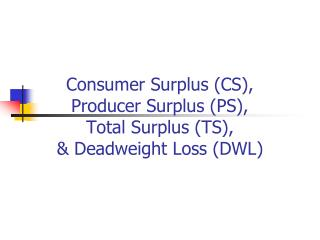DownloadDownload PresentationConsumer Surplus (CS), Producer Surplus (PS), Total Surplus (TS), & Deadweight Loss (DWL)

# Consumer Surplus (CS), Producer Surplus (PS), Total Surplus (TS), & Deadweight Loss (DWL)

Download Presentation## Consumer Surplus (CS), Producer Surplus (PS), Total Surplus (TS), & Deadweight Loss (DWL)

- - - - - - - - - - - - - - - - - - - - - - - - - - - E N D - - - - - - - - - - - - - - - - - - - - - - - - - - -
##### Presentation Transcript

1. Consumer Surplus (CS), Producer Surplus (PS), Total Surplus (TS),& Deadweight Loss (DWL)

2. Do You Know How to Calculate on a Graph …? • Consumer Surplus (CS) • Producer Surplus (PS) • Total Surplus (TS) • Deadweight Loss (DWL)

3. CS, PS, TS, & DWL \$ Given the D & S curves in a market as shown, 12 10 S 8 6 4 2 D 200 400 600 Q

4. CS—The Benefits to Buyers (Equivalent to Bargain/Saving) \$ CS is the shaded triangle. Its value equals the area ofthe triangle, or half or a rectangle. The formula is: area = base x ½ height.So CS = (300 x ½ x (12-6)) = \$900. 12 10 S 8 6 4 2 D 200 400 600 Q

5. PS—The Benefits to Sellers(Equivalent to Gross Profit) \$ PS is the shaded triangle. Its value equals the area ofthe triangle.So PS = base x ½ height = (300 x ½ x (6-2)) = \$600. 12 10 S 8 6 4 2 D 200 400 600 Q

6. TS = CS + PS = Maximum(TS Is Maximized in a Free Market) \$ In a free market, TS, the sum of CS and PS, measures the total maximum benefits to all market participants. TS = CS + PS = \$900 + \$600 = \$1,500. 12 10 S 8 6 4 2 D 200 400 600 Q

7. DWL \$ Given the D & S curves, suppose that the government imposed a per-unit tax (T) on the market as shown. What would happen? 12 10 S 8 6 4 2 D 200 400 600 Q

8. TS = CS + PS < Maximum (DWL Is the Loss/Reduction in TS) \$ CS would decrease; PS would decrease; GTR would rises, TS would decrease; and a DWL would exist. 12 CS aftertax 10 S 8 GovernmentTax Revenue(GTR) DWL GTR = \$3 x 200 = \$600; andDWL = ½ x 3 * 100 = \$150 6 PS aftertax 4 2 D 200 400 600 Q

9. Now You Know How to Calculate on a Graph … • Consumer Surplus (CS) • Producer Surplus (PS) • Total Surplus (TS) • Deadweight Loss (DWL)

10. See You! Take Care!